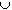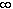#Interactive Real Analysis

Next | Previous | Glossary | Map

## 1.1. Notation and Set Theory

### Examples 1.1.2(b):

If A = {xR : -4 < x < 3} and B = {xR : -1 < x < 7}, then find AB, AB, A \ B, and comp(A).
AB = { xR : -4 < x < 7 }AB = { xR : -1 < x < 3 }A \ B = { xR : -4 < x-1 }comp( A ) = { xR : -< x-4 }{ xR : 3x <}Next | Previous | Glossary | Map